# Asian option valuation

Asian options differ in payoff calculation from European and American option. The payoff for the latter is just the difference between the spot price minus the strike price (call). The payoff for an Asian option equals the average price realized minus the strike (call) or the strike minus the average price realized.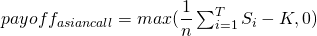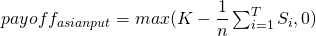## Asian option valuation: simulation ingredients

Due to the odd payoff of an Asian option, it can not be valued through an analytical formula. However they can be valued through a simulation experiment. In many simulation exercises, the geometric Brownian motion, as shown below, can be used to model the underlying stock behaviour. In this formula S equals the price of the stock, μ equals the stock’s return, σ equals the stock’s volatility and Δt equals 1 time step.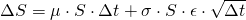In order to implement the stock price evolution in Excel this has to be restated as follows: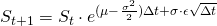With an uncertainty parameter ε generated by a certain distribution, often just a normal distribution.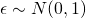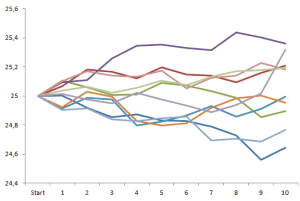## Asian option valuation: simulation implementation

The value of an Asian option can in practice be determined based on the following method:

Step 1: Determine the return μ, the volatility σ, the risk free rate r, the time horizon T and the time step Δt

Step 2: Generate using the formula a price sequence

Step 3: Calculate the payoff of the Asian call and or put and store it

Step 4: Apply step 2 and 3 N times (e.g. 10000)

Step 5: Calculate the average of all the stored payoffs

Step 6: Discount this value back to today

## Summary

Asian options are a special kind of option which shows a different behaviour than other less complex options. Due to their more complex nature, the value needs to be determined through simulations.

### Asian option valuation Excel implementation

Need to have more insights? Download our free excel file: Asian option valuation.